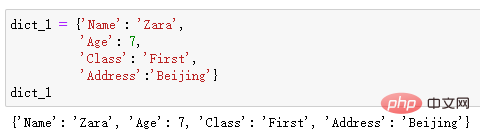# 三种方法使用For循环遍历Python字典（实例详解）`dict_1 = {'Name': 'Zara', 'Age': 7, 'Class': 'First','Address':'Beijing'}`## 方法 1：使用 For 循环 + 索引进行迭代

Python 会自动将`dict_1`视为字典，并允许你迭代其key键。然后，我们就可以使用索引运算符，来获取每个value值。

```for key in dict_1:
print(key, ":", dict_1[key])``````for key in sorted(dict_1):
print(key, ":", dict_1[key])```## 方法 2：使用 .keys( ) + 索引进行迭代

```for key in dict_1.keys():
print(key, '-->', dict_1[key])```## 方法 3：使用 .items( ) 进行迭代

`print(dict_1.items())````for k,v in dict_1.items():
print(k,">>",v)``````for a,b in dict_1.items():
print(a,"-",b)```## 进阶：遍历嵌套字典

```dict_2 = {"num_1":{'Name': 'Zara', 'Age': 7, 'Class': 'First','Address':'Beijing'},
"num_2":{'Name': 'BOb', 'Age': 32, 'Class': 'Six','Address':'Shanghai'},
"num_3":{'Name': 'Tom', 'Age': 25, 'Class': 'Second','Address':'Wuhan'}}dict_2``````for k, v in dict_2.items():
if type(v) is dict:
for nk, nv in v.items():
print(nk, "→", nv)``````for k, v in dict_2.items():
if type(v) is dict and k == 'num_2':
for sk, sv in v.items():
print(sk, "-->", sv)```1/1